Courses

# Properties of Determinants - Matrices and Determinants, Business Mathematics & Statistics B Com Notes | EduRev

## B Com: Properties of Determinants - Matrices and Determinants, Business Mathematics & Statistics B Com Notes | EduRev

The document Properties of Determinants - Matrices and Determinants, Business Mathematics & Statistics B Com Notes | EduRev is a part of the B Com Course Business Mathematics and Statistics.
All you need of B Com at this link: B Com

## Properties of Determinants And Some Important Determinants to Remember

### Properties of Determinants:-

1. Determinant of a matrix is same as the determinant of its transpose.
2. If two rows or columns of a determinant are interchanged the determinant changes its sign.
3. If the elements of a row (column) of a determinant are multiplied by a constant K, then the determinant will be multiplied by the same constant. For example,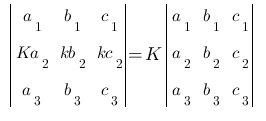If A is a square matrix of order n, then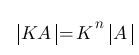4.If all the elements of a row (column) of a determinant are zeros, then the value of that determinant is also zero.

5.If two rows (columns) of a determinant are equal then value of that determinant is zero.
Note:- If two rows (2 columns) of a determinant are proportionate then its value is zero.
6.If the elements of a row (column) of a determinant are sums of two elements then the determinant can be expressed as the sum of two determinants. That is for example,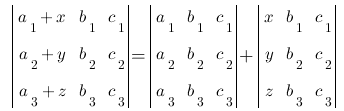7. If the elements of a row (column) of a determinant are added to or subtracted from the corresponding elements of some other row (column) then the determinant remains unchanged.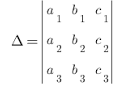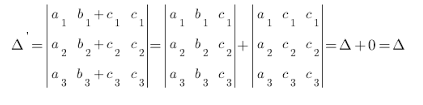8. If the products of the elements of a row (or column) of a determinant with a constant K are added to the corresponding elements of some other row (or column), then the determinant remains unchanged.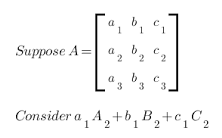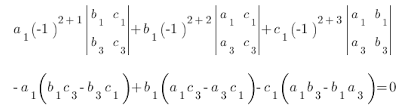9. Sum of the products of the elements of a row in a square matrix and the co-factors of the corresponding elements of some other row (column) is zero.
10. If the rows or columns of a determinant are changed without disturbing a cyclic order, then the determinant remains unchanged. That is,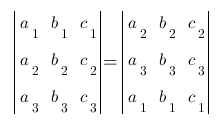11. Determinant of a null matrix is 1.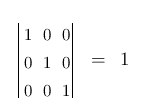12. Determinant of a null matrix of the order 3X3 is zero.
Some Important Matrices Determinants to be Remembered for competitive exams:-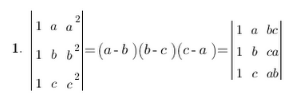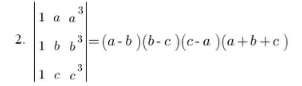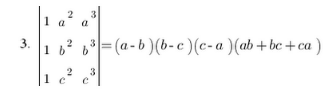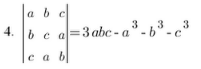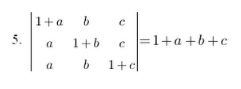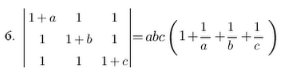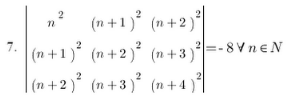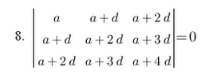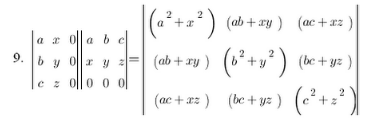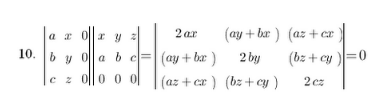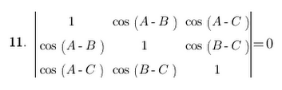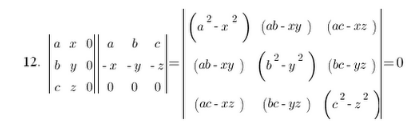The document Properties of Determinants - Matrices and Determinants, Business Mathematics & Statistics B Com Notes | EduRev is a part of the B Com Course Business Mathematics and Statistics.
All you need of B Com at this link: B ComUse Code STAYHOME200 and get INR 200 additional OFF Use Coupon Code
All Tests, Videos & Notes of B Com: B Com122 videos|142 docs

Track your progress, build streaks, highlight & save important lessons and more!

,

,

,

,

,

,

,

,

,

,

,

,

,

,

,

,

,

,

,

,

,

,

,

,

;